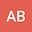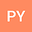••Pressure-to-depth conversion is a crucial step towards geodynamic reconstruction but remains strongly debated. Here, we derive pressure-to-depth conversion models using either one or two pressure data points in conjunction. In the two-point method, we assume that both peak and retrograde pressure are recorded at the same depth. This method reduces the depth estimate uncertainty dramatically. We apply the proposed pressure-to-depth conversions to a large set of $P$ data from (ultra)high-pressure metamorphic rocks. We explore different cases to explain the transition from peak to retrograde pressure by varying the direction and magnitude of stress components. Our results show that (1) even small deviatoric stresses have a significant impact on depth estimates, (2) the second principal stress component $\sigma_2$ plays an essential role, (3) several models can explain the $P$ evolution of the data but lead to different depth estimates, and (4) strain data offer a means to falsify two-point models. The most commonly used pressure-to-depth conversion method uses one pressure point and the assumption that pressure is lithostatic. Then, the transition from peak to retrograde pressure is interpreted as the result of deep subduction ($>100$ km), followed by fast exhumation to mid-crustal depth. We show that alternative models where a change in the stress state at a constant depth triggers the pressure transition explain the data equally well. The predicted depth is then shallower than the crustal root Moho ($<75$ km) for all data points.